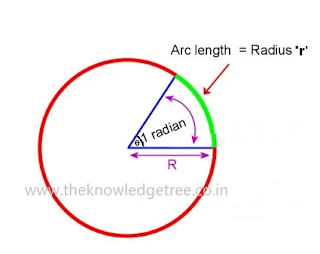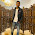## Mathematical tools everyone should know

ANGLE: Amount of revolution of a given line about a fixed line is called theFig.1: Angle and Quadrants
angle traced by the line.

● Angle taken as positive when rotation is in the anticlockwise direction.

●Negative, when taken in the clockwise direction.
(For angle rotation everyone should have an idea of quadrant.)
● Angle can be measured in degrees or radians.
Sexagimal System (degree)
Right angle = 90 °
1° =60' (60 minutes)
1'=60'' (60 seconds)
1' is the 60th division if 1°and 1" is the 60th division of x'. It should not be confused with minutes and seconds of a clock.

CIRCULAR SYSTEM
One radian is the angle subtended at the center of a circle by an arc equal in length to the radius of the circle as in fig.2.
Hence, length of the arc’s’ is related to angle in radians subtended at the center of the circle by this arc asFig.2.
S = r θ               (θ called theta)

● Since, angle subtended by a complete circle at the center in radians will be
θ = S / r = 2πr / r (angle subtended by a complete circle).
θ = 2π
1 radian= 360°/2π = 180°/ π = 57.27°

Conversion of some angles from degrees into radians
1. 30° degree
Degree = radian (just for students understanding)
30 = 180°/π
30 × π / 180= π/6
= 2. 45°Fig.3. Radian vs Degree
π / 180×45 = π / 4

Do yourself for exercise 60°,90°,120°, 135°, 180°, 210°,300°, 360°
Conversion of some angles from radians into degrees:
1. 2π/3 = 2/3×180°=120°
2. π/4 = 1/4× 180° = 45°

Do yourself for exercise 3 π / 2

Trigonometry Ratios:
Perpendicular side always be opposite to angle θ. And base is always opposite to right angle as in fig.4.
We all have basic idea about T-ratios; let’s discuss briefly.
 Fig.5. T-Ratios
Value of T-ratio at Standard angle.fig. Values of T-ratio

Allied angle is any angle which can be written as 90° × n ± θ    where 'n = 0, 1, 2,.....'

Two special of right angle triangle as in fig.Fig. two special case of right angle triangle
note : Students can use direct values of T-ratio if they have triangles with there.

•  Some addition and subtraction formulae of Trigonometry
1. Sin( A + B) = sin A c os B sin B cos A

2. Sin( A -- B )  = sin A cos B --     sin B cos A

3. Cos (A + B) = cos A cos B - sin A sin B

4. Cos(A - B) = cos A cos B + sin A sin B
• C - D Formulae
1. Sin C + Sin D = 2 Sin[(C+D)/2]Cos[(C-D)/2]
2. SinC - SinD = 2 Cos[(C+D)/2]Sin[(C-D)/2]
3. CosC + CosD = 2 Cos[(C+D)/2]Cos[(C-D)/2]
4. CosC - CosD = 2 Sin[(C+D)/2]Sin[(D-C)/2]
for e.g
1. Sin 75° = ?
ans. Sin 75° = Sin (45° + 30°)
using sin( A + B) = sin A c os B  sin B cos A
Sin 45° cos 30° + cos 45° sin 30°
using Value of T-Ratio
= (1/√2 ) (√3/2) + (1/√2) (1/2)
= [(√3 + 1 ) / (2√2)]
Trigonometry ratio of multiple angle & Identity
1.  Cos2θ = Cos²θ - Sin²θ
= 2Cos²θ - 1
= 1 - 2Sin²θ
(1-tan²θ)/(1+tan²θ)
2. Sin2θ = 2SinθCosθ = (2tanθ)/(1+tan²θ)
3. Sin²θ + Cos²θ = 1
4. Tan²θ + 1 = Sec²θ
5. Cot²θ + 1 = Cosec²θ

To Be Conti..... in part - 2

References: NCERT books, Moderns pub., Internet Sources

1.Great.

2.Easy way to explain your contant.
Very good....

3.Thanks and keep Supporting

4.Well done...

5.6.Great ������������

7.Well done brother..

8.It's really hoping.thx sir

9.It's really helping.thx sir

10.Thx and Keep Supporting. And also you can send your query here in comment box or from above tab "contact us"

11.Thx and Keep Supporting. And also you can send your query here in comment box or from above tab "contact us"